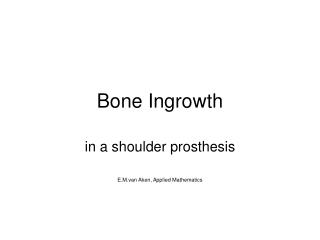# Bone Ingrowth - PowerPoint PPT PresentationDownload PresentationBone Ingrowth

Bone Ingrowth
Download Presentation## Bone Ingrowth

- - - - - - - - - - - - - - - - - - - - - - - - - - - E N D - - - - - - - - - - - - - - - - - - - - - - - - - - -
##### Presentation Transcript

1. Bone Ingrowth in a shoulder prosthesis E.M.van Aken, Applied Mathematics

2. Outline • Introduction to the problem • Models: • Model due to Bailon-Plaza: Fracture healing • Model due to Prendergast: Prosthesis • Numerical method: Finite Element Method • Results • Model I: model due to Bailon-Plaza -> tissue differentiation, fracture healing • Model II: model due to Prendergast -> tissue differentiation, glenoid • Model II: tissue differentiation + poro elastic, glenoid • Recommendations

3. Introduction • Osteoarthritis, osteoporosis  dysfunctional shoulder • Possible solution: • Humeral head replacement (HHR) • Total shoulder arthroplasty(TSA): HHR + glenoid replacement

4. Introduction

5. Introduction • Need for glenoid revision after TSA is less common than the need for glenoid resurfacing after an unsuccesful HHR • TSA: 6% failure glenoid component, 2% failure on humeral side

6. Model

7. Model • Cell differentiation:

8. Models • Two models: • Model I: Bailon-Plaza: • Tissue differentiation: incl. growth factors • Model II: Prendergast: • Tissue differentiation • Mechanical stimulus

9. Model I • Geometry of the fracture

10. Model I • Cell concentrations:

11. Model I • Matrix densities: • Growth factors:

12. Model I • Boundary and initial conditions:

13. Finite Element Method • Divide domain in elements • Multiply equation by test function • Define basis function and set • Integrate over domain

14. Numerical methods • Finite Element Method: • Triangular elements • Linear basis functions

15. Results model I After 2.4 days: After 4 days:

16. Results model I After 8 days: After 20 days:

17. Model II • Geometry of the bone-implant interface

18. Model II • Equations cell concentrations:

19. Model II • Matrix densities:

20. Model II • Boundary and initial conditions:

21. Model II Proliferation and differentiation rates depend on stimulus S, which follows from the mechanical part of the model.

22. Results Bone density after 80 days, stimulus=1

23. Results

24. Model II Poro-elastic model • Equilibrium eqn: • Constitutive eqn: • Compatibility cond: • Darcy’s law: • Continuity eqn:

25. Model II • Incompressible, viscous fluid: • Slightly compressible, viscous fluid:

26. Model II Incompressible: Problem if Solution approximates Finite Element Method leads to inconsistent or singular matrix

27. Model II Solution: 1. Quadratic elements to approximate displacements 2. Stabilization term

28. Model II • u and v determine the shear strain γ • p and Darcy’s law determine relative fluid velocity

29. Model II Boundary conditions

30. Results Model II Arm abduction 30 ° Arm abduction 90 °

31. Results Model II 30 ° arm abduction, during 200 days

32. Results Model II Simulation of 200 days: first 100 days: every 3rd day arm abd. 90°, rest of the time 30 °. 100 days200 days

33. Recommendations • Add growth factors to model Prendergast • More accurate simulation mech. part: • Timescale difference between bio/mech parts • Use the eqn for incompressibility (and stabilization term) • Extend to 3D (FEM)

34. Questions?